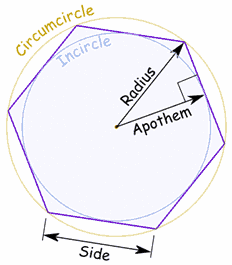## Regular polygon area calculator

 Apothem(radius of inscribed circle) No.of Sides of Polygon Length of each side Unit FeetInchesMeterCenti MeterMilli Meter
 Result: Area of Polygon Unit

Regular Polygon Calculator to find out the Polygon Area when given number of polygon sides, values of apothem, and length of each side.

### Regular Polygon area formulaRectangle Polygon Area A = (1/2) Apothem x No. of Sides x Length of side

Thinkcalculator.com provides you helpful and handy calculator resources.